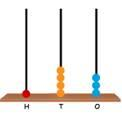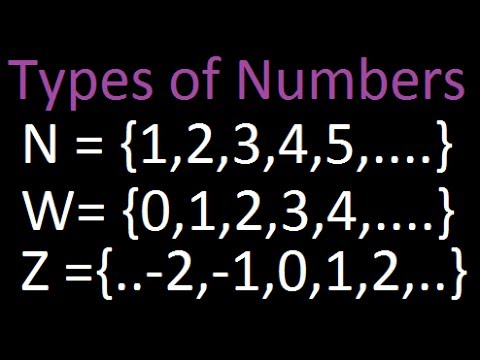Courses

# Test: 3-Digit Numbers- 1

## 10 Questions MCQ Test Mathematics for Class 2 | Test: 3-Digit Numbers- 1

Description
This mock test of Test: 3-Digit Numbers- 1 for Class 2 helps you for every Class 2 entrance exam. This contains 10 Multiple Choice Questions for Class 2 Test: 3-Digit Numbers- 1 (mcq) to study with solutions a complete question bank. The solved questions answers in this Test: 3-Digit Numbers- 1 quiz give you a good mix of easy questions and tough questions. Class 2 students definitely take this Test: 3-Digit Numbers- 1 exercise for a better result in the exam. You can find other Test: 3-Digit Numbers- 1 extra questions, long questions & short questions for Class 2 on EduRev as well by searching above.
QUESTION: 1

### Which of the following number is smaller than 156?

Solution:

89 comes before 156.
Hence, 89 is less than 156.

QUESTION: 2

### Which of the following shows abacuses placed in the ascending order?

Solution:

134 < 156 <176 <192. The abacuses show number 134, 156, 176 and 192 in ascending order.
As they are placed from smallest to greatest.

QUESTION: 3

### Which of the following shows abacuses placed in descending order?

Solution:

173 > 172 > 162 > 126
As the numbers are arranged from greatest to smallest.
The abacuses show the numbers 173, 172, 162 and 126 in descending order.

QUESTION: 4

Which of the following numbers is greater than 189?

Solution:

198 comes after 189.
Hence, 198 is greater than 189.

QUESTION: 5

Which of the following number is equal to 145?

Solution:

145 is equal to 145.

QUESTION: 6

Which of the following number is greater than 197?

Solution:

198 comes just after 197.
Hence, 198 is greater than 197.

QUESTION: 7

Which of the following number is smaller than 165?

Solution:

156 comes before 165.
Hence, 156 is smaller than 165.

QUESTION: 8

Which of the following number is greater than the number shown on the abacus?Solution:

The number shown on the abacus is 143. 154 comes after 143.
Hence, 154 is greater than 143.

QUESTION: 9

Riya writes a number greater than 176 in her notebook. She erased the last digit of that number. The two-digit number formed after erasing the last digit is 17. Which of the following numbers did she erase in the ones place of that number?

Solution:

The first two digits of the three-digit number that Riya wrote is 1 and 7.
The number written by Riya is greater than 176.
When we write 8 as the last digit after 17, we get 178.
178 is greater than 176.
So, the digit at the ones place of the number was 8.
Hence, Riya erased 8 by mistake.

QUESTION: 10

Which of the following shows numbers arranged in ascending order?

Solution:

In ascending order the numbers are arranged from smallest to greatest.
Hence, 113 < 124 < 128 < 129 are arranged in ascending order.

Track your progress, build streaks, highlight & save important lessons and more!

### Similar Content### Related tests